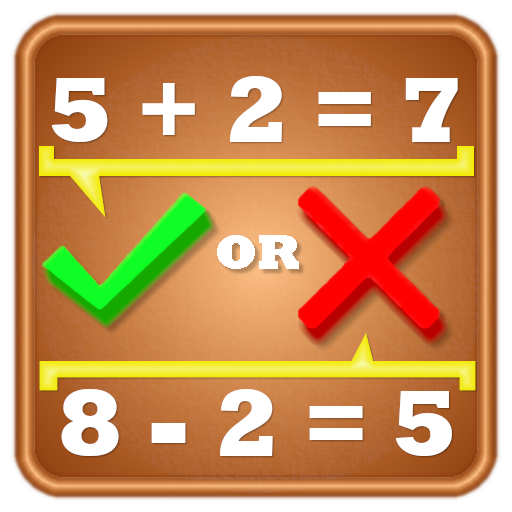## True or False - Free Math Game

### by Rohit Porwal

Free
1304
Categories Tags
###### Description

True or false math game is the best game to train your brain to solve math problems faster.

In this math game, you need to identify whether the given equation is True or False.

For this first you have to solve the mathematical equation, and then you have to compare the result with the result given in equation and answer whether the given equation is true or false.
And all this you have to do in a short period of time.

So this game improves two of your brain functions:
1) Calculation skills
2) Recognition and comparing abilities.

So, what this game offers you:
1) Game:
It covers math question from following topics:
b) Subtraction
c) Multiplication
d) Division
e) Squares and square roots
f) Cubes and cube roots

There are three modes of game:
i) Easy Mode - Easy math questions of type addition, subtraction, multiplication and division.

ii) Hard Mode - Tough math questions covering all topics and higher difficulty level.

iii) Rapid Fire mode - Questions are easy, but you have only two seconds to answer each question.

2) Challenges: There are 300 levels of challenges in this game.
These challenges focus on your speed and accuracy.
Difficulty level increases with number of levels.

3) Duel Game - You can compete with your friends on the same device.

4) Practice: It provides you personalized practice sessions where you can set number of questions and type of questions.

Apart from all this you can customize your view with the color of your choice and language you are comfortable with.

• GOTD

2471

1869

1668